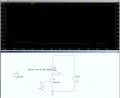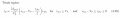More accurate JFET model for DMM input?

DarthVolta

Joined Jan 27, 2015
521
This is 1 of the input paths, of a DMM, when 1k+10M+1.111M, are over a common source JFET, that's something high imp. like a 2n4119A (called J2715 but can't find pdf, seems it's not off the shelf...I'll have to pull one and curve trace)

Using the Beta, Vp, and Lamda in LTSpice (listed below) I can use the typical linear or saturation region JFET eqn's and get a close enough answer for most stuff, expect in a case where Rd is so high, that even the Idss will swamp the Rd*Id to the point that Vds is near zero.

For instanceSo I tired using 2 versions of the Linear region eqn
w/ Vgs=0, Vdd=10V, B=22uS/V, Lamda=10m/V, Vp=-4.5V
Rd=11.112Meg

Id= β⋅Vds⋅[2⋅(Vgs-Vp)⋅Vds]⋅(1+λ⋅Vds)
for 0<Vds<Vgs-Vp

andSo in the 1st using Vds=Vdd-RdId, you end up with a big cubic eqn, than fails giving Id1,2,3 =12.82uA, -561nA , -561nA (on my TI36Xp when working out A,B,C,D seperate and plugging that in)

And w/ the 2nd, quadratic eqn I get 111.2nA and 729.6nA

And LTSice using a more accurate model gets 889.81nA

When using BJT's, I'm used to Vce going down to 0.2V, and I soon I'm sure I'll notice how off that can be, for basic models.

So what should you do when the Vdd, Rd and Idss values mean Vds is getting squeezed to 0V ? Is there anything special to do when Vds is nearing zero ? I'm ignoring little rd rs of the channel, making Vds=0 does not work in the eqn's of course, maybr LHR and limits help.

Last edited: